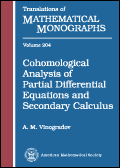### Cohomological Analysis of Partial Differential Equations and Secondary Calculus

By: Alexandre Vinogradov, University of Salerno, Italy.

Translations of Mathematical Monographs, vol. 204, American Mathematical Society, 2001, 247 pages, ISBN: 0-8218-2922-X, ISSN: 0065-9282.

Description

This book is dedicated to fundamentals of a new theory, which is an analog of affine algebraic geometry for (nonlinear) partial differential equations. This theory grew up from the classical geometry of PDE's originated by S. Lie and his followers by incorporating some nonclassical ideas from the theory of integrable systems, the formal theory of PDE's in its modern cohomological form given by D. Spencer and H. Goldschmidt and differential calculus over commutative algebras (Primary Calculus). The main result of this synthesis is Secondary Calculus on diffieties, new geometrical objects which are analogs of algebraic varieties in the context of (nonlinear) PDE's.

Secondary Calculus surprisingly reveals a deep cohomological nature of the general theory of PDE's and indicates new directions of its further progress. Recent developments in quantum field theory showed Secondary Calculus to be its natural language, promising a nonperturbative formulation of the theory.

In addition to PDE's themselves, the author describes existing and potential applications of Secondary Calculus ranging from algebraic geometry to field theory, classical and quantum, including areas such as characteristic classes, differential invariants, theory of geometric structures, variational calculus, control theory, etc. This book, focused mainly on theoretical aspects, forms a natural dipole with Symmetries and Conservation Laws for Differential Equations of Mathematical Physics, Volume 182 in this same series, Translations of Mathematical Monographs, and shows the theory "in action".

Contents
• From symmetries of partial differential equations to Secondary Calculus
• Elements of differential calculus in commutative algebras
• Geometry of finite-order contact structures and the classical theory of symmetries of partial differential equations
• Geometry of infinitely prolonged differential equations and higher symmetries
• C-spectral sequence and some applications
• Introduction to Secondary Calculus
• Bibliography
• Index

• List Price: \$89
• Institutional Member Price: \$71
• Individual Member Price: \$53
• Order Code: MMONO/204